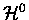The Born method  extends the Born-Oppenheimer approximation by including corrections to the effective potential E0(R) in Eq. 2.4. The full Hamiltonian, separated in two parts, includes the termdescribing the relative motion of the two nuclei as well as the coupling between the electronic (muonic) and the nuclear motions, in addition to the termfor the fixed nuclear problem;(19)

Note thatincludes nuclear repulsion in addition to the electronic interaction with the nuclei. The total wave function is expanded in the complete set of:(20)

whereare the eigenstates of the fixed nuclei problem Eq. 2.3. The solution to the problem(21)

is obtained by substituting (2.6) into (2.5), multiplying the result by, and integrating over r, which yields(22)

where(23)

Note that in (2.8), the diagonal termis moved to the left-hand side, hence the summation is for off-diagonal terms (). In the Born adiabatic approximation, the right hand side of (2.8), i.e. the coupling of different electronic states m, is neglected, leaving only the first term in the expansion in Eq. (2.6). Thus, the equation for nuclear motion reads(24)

with the effective potential for a given electronic state n given by(25)

which includes the diagonal correction to the zero order potential, taking partly into account the coupling between the nuclear and electronic (muonic) motion. The Born-Oppenheimer approximation, on the other hand, neglects this coupling, and assumes U'n (R) = E 0n (R) ,as was seen in Eq. 2.4.

The validity of the Born-Oppenheimer approximation is dependent on the smallness of the expansion parameterwhereis the reduced mass of the two nuclei, and m the mass of electron or muon. The parameterreflects the ratio of the amplitudes of the nuclear motion in comparison to the equilibrium internuclear distance, hence the assumption thatallows one to neglect the termin (2.8), which contains derivatives ofwith respect to R . Dependence of the Born approximation onis less clear and somewhat qualitative, and the accuracy depends on specific characteristics of the problem including the strength of off-diagonal coupling in Eq. 2.8.

In the case of the muonic molecule,is not small. For its loosely bound states, which are our main interest, the vibrational amplitudes are not small compared to the internuclear distance. Hence both the Born-Oppenheimer and the Born Adiabatic approximation fail to describe even qualitative characteristics of the muonic molecule, such as the number of the bound states . As for loosely bound states, the former method gives a state bound much too deeply, while with the latter method the state is not bound at all .